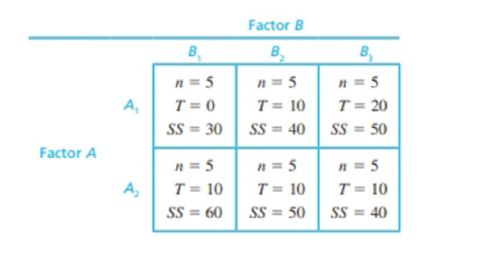Chapter 13.3, Problem 2LCAEssentials of Statistics for the B...

8th Edition
Frederick J Gravetter + 1 other
ISBN: 9781133956570

Solutions

Chapter
SectionEssentials of Statistics for the B...

8th Edition
Frederick J Gravetter + 1 other
ISBN: 9781133956570
Textbook Problem

The following data summarize the results from a two-factor independent-measures experiment:a. Calculate SS for factor A.b. Calculate SS for factor B.c. Given that the between-treatments (or between-cells) SS is equal to 40, what is the SS for the interaction?

a.

To determine

To find: The SS for factor A

Explanation

Given info:

The summarized data of the two-factor independent measures experiment.

Calculation:

The formula for SSA is

SSA=Trow2nrowG2N

Calculating the <

b.

To determine

To find: The SS for factor B

c.

To determine

To find: SS for interaction

Still sussing out bartleby?

Check out a sample textbook solution.

See a sample solution

The Solution to Your Study Problems

Bartleby provides explanations to thousands of textbook problems written by our experts, many with advanced degrees!

Get Started

Find the mean, median, and mode for the following scores; 8, 7, 5, 7, 0, 10, 2, 4, 11, 7, 8, 7

Essentials of Statistics for The Behavioral Sciences (MindTap Course List)

24. (a) Graph . (b) Graph . (c) Algebraically show why these graphs are identical.

Mathematical Applications for the Management, Life, and Social Sciences

The area of the parallelogram at the right is: 26

Study Guide for Stewart's Multivariable Calculus, 8th

The radius of convergence of is: 1 3 ∞

Study Guide for Stewart's Single Variable Calculus: Early Transcendentals, 8th